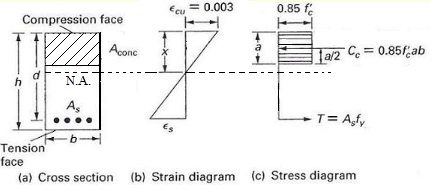Singly Reinforced Concrete Beam

Analysis of Singly reinforced concrete beam

Concrete is weak in tension therefore steel is added in tension zone of the section to provide strength in tension. The figure given below shows a typical section of singly reinforced concrete beam with strain distribution and equivalent stress distribution (proposed by Whitney).The actual stress distribution of concrete is parabolic. Whitney (1930) proposed an equivalent rectangular stress distribution, which is adopted by ACI - 318. This rectangular stress block has  made the calculations easy. It has an average stress of 0.85fC'  and the depth "a" equal to β1  times neutral axis of section. The value of β1  is taken as 0.85 for fC'  upto 4000  psi (30 MPa), and 0.05 less for each 1000 psi (7 MPa) of fC'   in excess of 4000 psi (30 MPa), but not less than 0.65.

The nominal moment strength is obtained by applying static equilibrium and compatibility of stress and strain. The design strength is calculated by dividing nominal strenght by strength reduction factor φ (phi).

With reference to the equivalent stress diagram as per Whitney,

Force of tension due to tension rebar;

T = (area tension rebar) * (stress of steel)

T =  As fy

It is required that tension steel has yielded when failure takes place. This makes sure that the section is not controlled by concrete.

Foce of compression (due to concrete);

Cc = (average compressive stress) * (equivalent area of concrete)

Cc = (0.85fC' ) * (b a)

For equilibrium, the force of tension equals the force of compression.This gives the values of "a";

a = (As fy ) / (0.85fC' b)

The depth of neutral axis; x = a/β1

The nominal moment strength Mn of the section is calculated by taking the moment of compressive force about the centroid of tension steel area.

Mn = Cc (d - a/2)

effective depth "d" is the distance from extreme compression fibre to the centroid of tension rebar.  Do you want to know what is doubly reinforced Concrete beam?

You can also use our free online calculator for strength of Reinforced Concrete beam.

#### Excellent Calculators

Stress Transformation Calculator
Calculate Principal Stress, Maximum shear stress and the their planes

To determine Absolute Max. B.M. due to moving loads.

Bending Moment Calculator
Calculate bending moment & shear force for simply supported beam

Moment of Inertia Calculator
Calculate moment of inertia of plane sections e.g. channel, angle, tee etc.

Reinforced Concrete Calculator
Calculate the strength of Reinforced concrete beam

Moment Distribution Calculator
Solving indeterminate beams

Deflection & Slope Calculator
Calculate deflection and slope of simply supported beam for many load cases

Fixed Beam Calculator
Calculation tool for beanding moment and shear force for Fixed Beam for many load cases

BM & SF Calculator for Cantilever
Calculate SF & BM for Cantilever

Deflection & Slope Calculator for Cantilever
For many load cases of Cantilever

Overhanging beam calculator
For SF & BM of many load cases of overhanging beam

Civil Engineering Quiz
Test your knowledge on different topics of Civil Engineering

Research Papers
Research Papers, Thesis and Dissertation

List of skyscrapers of the world
Containing Tall buildings worldwide

Forthcoming conferences
Containing List of civil engineering conferences, seminar and workshops

Profile of Civil Engineers
Get to know about distinguished Civil Engineers

Professional Societies
Worldwide Civil Engineers Professional Societies